Courses

# Elements of Cache Design Computer Science Engineering (CSE) Notes | EduRev

## Computer Science Engineering (CSE) : Elements of Cache Design Computer Science Engineering (CSE) Notes | EduRev

The document Elements of Cache Design Computer Science Engineering (CSE) Notes | EduRev is a part of the Computer Science Engineering (CSE) Course Computer Architecture & Organisation (CAO).
All you need of Computer Science Engineering (CSE) at this link: Computer Science Engineering (CSE)

Cache size

• Size of the cache to be small enough so that the overall average cost per bit is close to that of main memory alone and large enough so that the overall average access time is close to that of the cache alone.
• The larger the cache, the larger the number of gates involved in addressing the cache.
• Large caches tend to be slightly slower than small ones – even when built with the same integrated circuit technology and put in the same place on chip and circuit board.
• The available chip and board also limits cache size.

6.6.2 Mapping function

• The transformation of data from main memory to cache memory is referred to as memory mapping process.
• Because there are fewer cache lines than main memory blocks, an algorithm is needed for mapping main memory blocks into cache lines.
• There are three different types of mapping functions in common use and are direct, associative and set associative. All the three include following elements in each example.
• The cache can hold 64 Kbytes
• Data is transferred between main memory and the cache in blocks of 4 bytes each. This means that the cache is organized as 16Kbytes = 214 lines of 4 bytes each.
• The main memory consists of 16 Mbytes with each byte directly addressable by a 24 bit address (224 = 16Mbytes). Thus, for mapping purposes, we can consider main memory to consist of 4Mbytes blocks of 4 bytes each.

Direct Mapping

• It is the simplex technique, maps each block of main memory into only one possible cache line i.e. a given main memory block can be placed in one and only one place on cache.
i = j modulo m
Where I = cache line number; j = main memory block number; m = number of lines in the cache
• The mapping function is easily implemented using the address. For purposes of cache access, each main memory address can be viewed as consisting of three fields.
• The least significant w bits identify a unique word or byte within a block of main memory. The remaining s bits specify one of the 2s blocks of main memory.
• The cache logic interprets these s bits as a tag of (s-r) bits most significant position and a line field of r bits. The latter field identifies one of the m = 2r lines of the cache.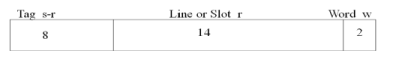• Address length = (s + w) bits
• Number of addressable units = 2s+w words or bytes
• Block size = line size = 2w words or bytes
• Number of blocks in main memory = 2s+ w/2w = 2s
• Number of lines in cache = m = 2r
• Size of tag = (s – r) bits
• 24 bit address · 2 bit word identifier (4 byte block)
• 22 bit block identifier
• 8 bit tag (=22-14), 14 bit slot or line
• No two blocks in the same line have the same Tag field
• Check contents of cache by finding line and checking Tag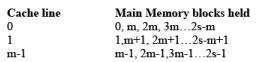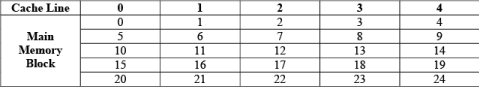Note that
* all locations in a single block of memory have the same higher order bits (call them the block number), so the lower order bits can be used to find a particular word in the block.
* within those higher-order bits, their lower-order bits obey the modulo mapping given above (assuming that the number of cache lines is a power of 2), so they can be used to get the cache line for that block
* the remaining bits of the block number become a tag, stored with each cache line, and used to distinguish one block from another that could fit into that same cache line.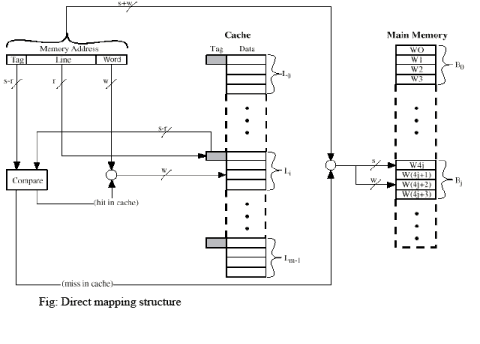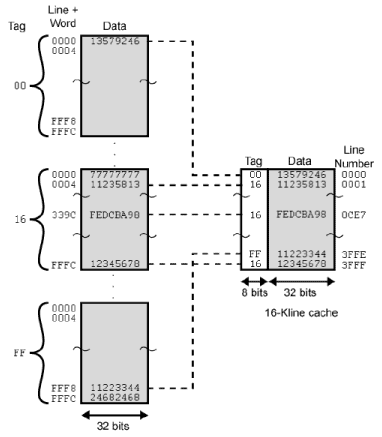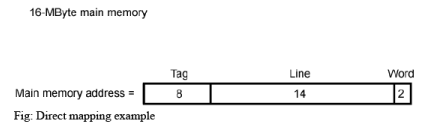Pros and Cons

• Simple
• Inexpensive
• Fixed location for given block
*  If a program accesses 2 blocks that map to the same line repeatedly, cache misses are very high

Associated Mapping

• It overcomes the disadvantage of direct mapping by permitting each main memory block to be loaded into any line of cache.
• Cache control logic interprets a memory address simply as a tag and a word field
• Tag uniquely identifies block of memory
• Cache control logic must simultaneously examine every line’s tag for a match which requires fully associative memory
• very complex circuitry, complexity increases exponentially with size
• Cache searching gets expensive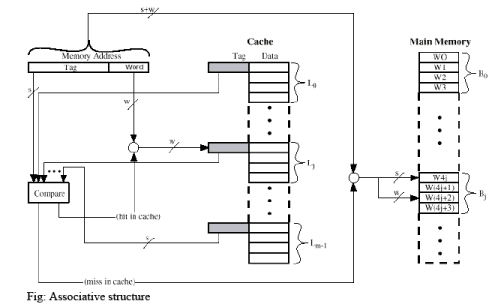• Address length = (s + w) bits
• Number of addressable units = 2s+w words or bytes
• Block size = line size = 2w words or bytes
• Number of blocks in main memory = 2s+ w/2w = 2s
• Number of lines in cache = undetermined, Size of tag = s bits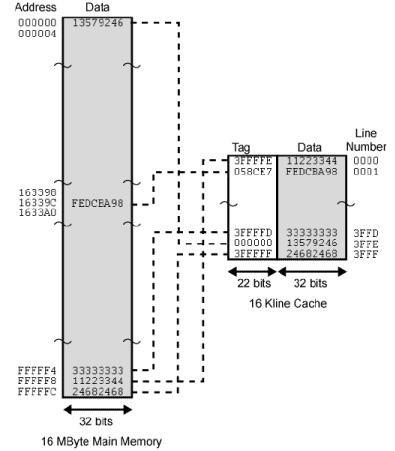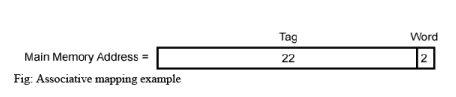• 22 bit tag stored with each 32 bit block of data
• Compare tag field with tag entry in cache to check for hit
• Least significant 2 bits of address identify which 16 bit word is required from 32 bit data block
• e.g.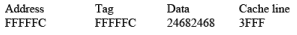Set Associated Mapping

• It is a compromise between direct and associative mappings that exhibits the strength and reduces the disadvantages
• Cache is divided into v sets, each of which has k lines; number of cache lines = vk M = v X k I = j modulo v Where, i = cache set number; j = main memory block number; m = number of lines in the cache
• So a given block will map directly to a particular set, but can occupy any line in that set (associative mapping is used within the set)
• Cache control logic interprets a memory address simply as three fields tag, set and word. The d set bits specify one of v = 2d sets. Thus s bits of tag and set fields specify one of the 2s block of main memory.
• The most common set associative mapping is 2 lines per set, and is called two-way set associative. It significantly improves hit ratio over direct mapping, and the associative hardware is not too expensive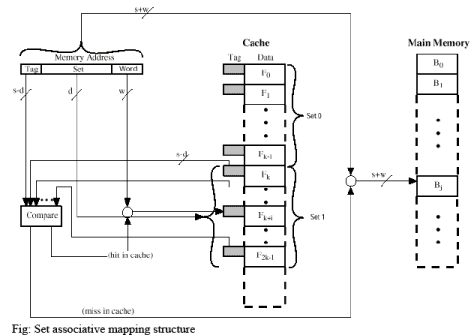• Address length = (s + w) bits
• Number of addressable units = 2s+w words or bytes
• Block size = line size = 2w words or bytes
• Number of blocks in main memory = 2
• Number of lines in set = k
• Number of sets = v = 2
• Number of lines in cache = kv = k * 2
• Size of tag = (s – d) bits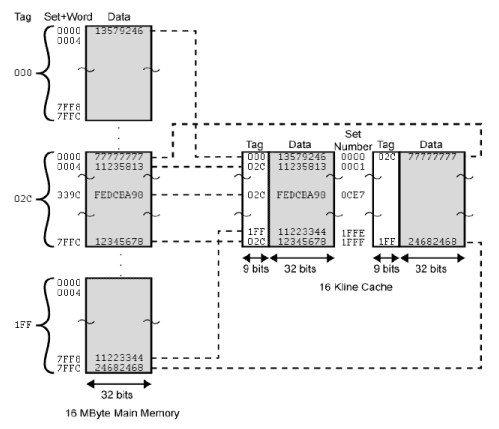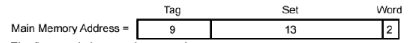Fig: Set associative mapping example

• 13 bit set number
• Block number in main memory is modulo 213
• 000000, 00A000, 00B000, 00C000 … map to same set
• Use set field to determine cache set to look in
• Compare tag field to see if we have a hit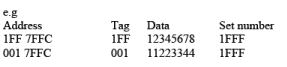Offer running on EduRev: Apply code STAYHOME200 to get INR 200 off on our premium plan EduRev Infinity!

## Computer Architecture & Organisation (CAO)

18 videos|63 docs|37 tests

,

,

,

,

,

,

,

,

,

,

,

,

,

,

,

,

,

,

,

,

,

;